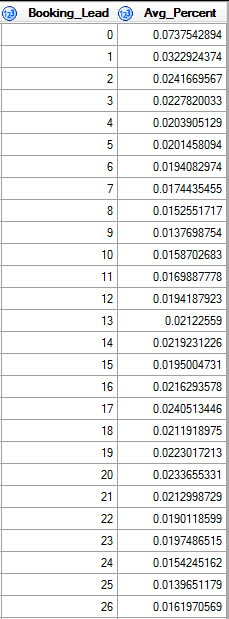## Draw Histogram and obtain 95% quantile verticle line

Hi,

I have a data set named A including two columns:I wuld like to draw histogram which reflects 'Booking_Lead' and its corresponding 'Avg_Percent'. Besides, I would like to draw a 90% quantile that starting from the largest 'Booking_Lead', and in this example, it is between 0 and 1, but near 1. And output the result called Table B.

I have tried the following:

ods graphics off;

proc univariate data=A noprint;
histogram Booking_Lead /BARLABEL=percent STATREF=P 90 STATREFLABEL="90th Pctl";
output out=B p90=p90pct;
run;

But this is not correct, How could I change the code, please?

Thank you!

3 REPLIES 3

## Re: Draw Histogram and obtain 95% quantile verticle line

It looks like your data is a summarization of the raw data. Does the avg_percent column sum to 1? Do you have the raw data?  If so, please post as a DATA step, not as an image file.

If you don't have the raw data, then compute the cumulative proportions by summing the avg_percent column:

data want;

set A;

cum_percent + avg_percent;

run;

The first observation for which cum_percent >= 0.9 is an estimate of the 90th percentile.

## Re: Draw Histogram and obtain 95% quantile verticle line

Hi Rick,

Thank you!  The 'Avg_Percent' column sum to 1. And my prior code to obtain this data is as below:

Proc Sql;
Create Table Work.A
as select distinct
Operating_Div_CD,
Chain_Cd,
LOC_ID,
avg(Percentage) as Avg_Percent
from Work.A_1
group by 1, 2, 3, 4
order by 4 desc;
Quit;

And I figured out the corresponding 'Booking_Lead' versus 90% quantile from your hint.

Thank you

## Re: Draw Histogram and obtain 95% quantile verticle line

The simpler way is to use

proc histogram data=A_1;

histogram Percentage;

etc.

Discussion stats
• 3 replies
• 785 views
• 1 like
• 2 in conversation